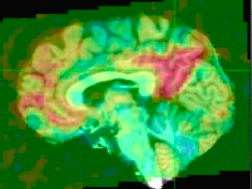# Weird timeseries produced for resting state fMRI

Dear experts,

I have preprocessed a resting state fMRI using afni_proc.py with the following command

``````
afni_proc.py -subj_id 003_S_6264 \
-blocks align tlrc volreg mask blur regress \
-anat_follower_erode vent wm \
-dsets /Volumes/WD_Elements/ADNI/fmri_afni/rsFmri.nii.gz -align_opts_aea -cost lpc+ZZ \
-volreg_align_e2a  -volreg_tlrc_warp  \
-tlrc_opts_at -init_xform CENTER \
-blur_size 5 -regress_polort 3 -regress_censor_outliers .1         \
-regress_censor_motion .2 -regress_apply_mot_types demean deriv      \
-regress_ROI_PC vent 1 -regress_ROI_PC wm 1 -regress_anaticor_fast   \
-regress_anaticor_label wm

``````

Everything went smoothly and also the connectivity map from the precuneus looks legit to me (see first figure attached)

However when I plot the time series thay look really weird and some time point is flat and the corresponding volume completely black (see second figure attached)

Any idea why and eventually how can i solve this?

Thanks

BestThe flat (all-zero volume) time points should correspond with those that are censored (due to outliers or motion, based on your options).

Those volumes are left in so that there is time correspondence between the original data and the residuals. Those volumes do not affect correlations within this dataset, but they could affect other things that one might do, such as correlations between different runs or subjects, or if running some ICA.

• rick

Dear Rick,

thanks for clarifying this, now makes much more sense. I was wondering whether if is there a way to interpolate those time points? In this way there will be time correspondence with the original data but without having those all-zero volumes?

Thanks a lot

So I understand, exactly what would be the purpose of adding data at those time points? That would alter correlations, perhaps unfairly. What further processing do you plan to do with this result?

Note that the proc script is probably using a 3dTproject command to generate the final errts dataset. It uses -cenmode ZERO to fill those censored time points with zeros. There is currently no option to alter that (i.e. to use NTRP instead of ZERO). But if would be possible to add an option for that, if it were useful.

• rick